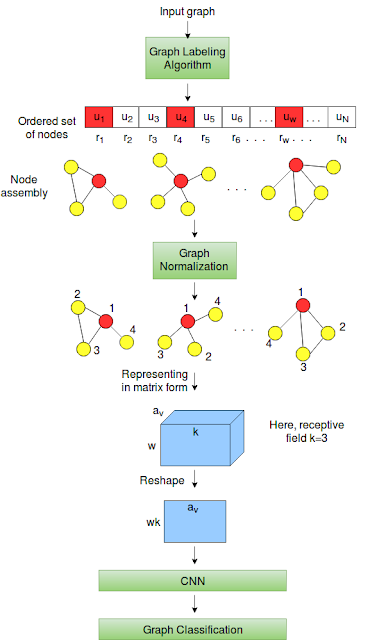### This post summarizes the PATCHY-SAN algorithm proposed by Niepert et al in their ICML 2016 paper "Learning Convolutional Neural Networks for Graphs"

• In the literature, the convolution operation on graphs is defined in 3 domains: spectral domain [Defferrard et al, Kipf et al], spatial domain [Masci et al, Boscaini et al] and embedding domain [Maron et al]. The method proposed by Niepert et al in "Learning Convolutional Neural Networks for Graphs" falls into the category of spatial domain algorithm.
• The broad aim of the paper is to learn a function over a bunch of graphs that will give us a general representation of them. These representations can then be used for any task such graph classification.
Challenges in applying convolution directly on graphs:
• There are two main problems that needs to be addressed before we could apply convolution on graphs.
• Challenge 1: Images can be thought of as a regular graph, where each pixel is denoted by a node and two adjacent pixels in the image can be connected by an edge. Similarly we can have regular graph over sentences. Data from these domains (images, text, speech) have spatial order. By spatial order, we mean that, given an image, there is first pixel, second pixel and so on i.e. it has unique order in which pixels are processed. However, that is not a case with graph structured data. The sequence in which nodes are processed is not unique.
• Challenge 2: The image data or text data (these well structured data is also called as data from Euclidean domain), has definite four neighbours-left pixel, right pixel, top pixel and bottom pixel. Hence, we could have a fixed size filter for all pixels irrespective of where it occurs in the image. However, a node in the graph can have many neighbours ranging from 1 to max degree of the graph and hence there is no specific left neighbour or top neighbour of a node. Because of varying number of neighbours, we can't use a one fixed size filter for all nodes. (Note that, degree distribution of graph follow long tail distribution, hence a naive approach of learning separate filters for each of node degree is not feasible solution).
PATCHY-SAN algorithm:Figure 1: PATCHY-SAN architecture.
• Step 1: Graph Labelling and Node Selection
• Niepert et al addresses the challenge 1 of not having unique node ordering by using graph labelling algorithm. It assigns label to each node in such a way that when we sort the nodes based on this label we get unique ordered set of nodes. Mathematically, it is a function $$l:V\rightarrow \hat{V}$$ i.e. it takes a set of nodes and outputs an ordered set of nodes.
• The graph labelling algorithm can be node degree, where the nodes with same degree will be assigned same label. It can be betweenness centrality or PageRank. The paper uses graph colouring algorithm called Weisfeiler-Lehman (WL) algorithm for graph labelling.
• For example, in above figure 1, there are total N nodes in the input graph, after applying graph labelling algorithm, we get an ordered set of nodes. This set is represented by an array. Let the highest rank node be represented by $$u_1$$ with rank $$r_1$$, second highest node denoted by $$u_2$$ with rank $$r_2$$ and so on.
• Once we have the ordered set of nodes, the algorithm selects $$w$$ nodes with a gap of stride $$s$$. In figure 1, from array of ordered set of nodes, the red colour boxes denote selected $$w$$ nodes These are selected with stride $$s$$=2.
• Step 2: Node Assembly
• The algorithm considers the neighbourhood subgraph of only the selected $$w$$ nodes for further processing. The neighbours are selected using breadth-first search algorithm.
• Step 3: Graph Normalization
• In images, each pixel has fixed number of neighbours and hence, the filter size is fixed. To use similar concept on graphs and address the challenge 2, the authors restrict the number of neighbourhood nodes considered for processing to value $$k$$ called as receptive field.
• If the neighbourhood nodes are more than $$k$$, then the relative ranking of neighbours wrt central node is considered to prune out the extra neighbours. For example, in figure 1, the receptive field $$k$$=3, the subgraph rooted at $$u_w$$ (right-most subgraph before and after the graph normalization step) had 4 neighbours, however, we keep only top 3 neighbours closest to root node $$u_w$$. The definition of closeness is given as: for a given central node $$u$$ and its two neighbourhood nodes $$v_i$$ and $$v_j$$, if $$dist(u,v_i)<dist(u,v_j)$$ implies $$rank(v_i)>rank(v_j)$$ and hence we keep $$v_i$$ and discard $$v_j$$.
• Finally, we have $$w$$ nodes with each having $$k$$ number of neighbours. This transformed graph data is represented in $$(w$$ x $$k$$ x $$a_v)$$ tensor, where $$w$$=number of selected nodes fro graph, $$k$$=fixed number of neighbours of each selected nodes and $$a_v$$=node feature vector. The rows $$w$$ of tensor is ordered according to label given by graph labelling algorithm in step 1.
• This tensor is reshaped to $$(wk$$ x $$a_v)$$ matrix, over which convolution operation is applied.
• The supervision for learning is provided by graph classification network.
Experiments:

• The algorithm was used for runtime analysis on four synthetic dataset. The features learnt by convolution layer are also used for visualization. The important experiment was of graph classification.
• The graph classification experiment is performed on 5 standard datasets: MUTAG, PTC, NCI-1, PROTEIN, D&D. The algorithm was also run on bigger social graphs for graph classification. Each dataset had around 12000 graphs with average 400 nodes in each graph.
• Experiments show that using convolution has actually improved the graph classification accuracy.
- Notes on Deep Geometric Matrix Completion for Recommendation Systems
- Summary of talk on Overview of Convolutional Neural Network for Graphs at workshop on New Deep Learning Techniques, IPAM UCLA

1.2.3.